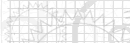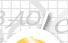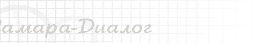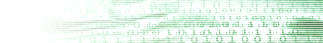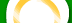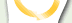| | | | | | |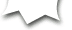POSTGRADUATE STUDYDegree-credit enrollment on specialities is open: 01.01.05 - probability theory and math. Statistics The supervisor of studies - Dr.Sci.Tech., professor Solodyannikov Y.V. 05.13.11 - mathematical and the software of computers, complexes and computer networks. Supervisors of studies - Dr.Sci.Phisics and Math., professor Blatov I.A. Dr.Sci.Tech., professor Mostovoy J.A. Dr.Sci.Tech., professor Solodyannikov Y.V. The program of entrance examination in postgraduate study on a speciality 05.13.11 - Mathematical and the software of computers, complexes and computer networks. 1. Vector spaces and linear mappings, the function, the operator, a functional. 2. The ordinary differential equations, examples of solutions. The equations in partial derivatives, examples of solutions, a method of characteristics. 3. Functions of complex variables, Fourier transform. 4. Main concepts of graph theory. A branch and bound algorithm. Dynamic programming. 5. Probability theory: discrete and arbitrary probability spaces, calculation of probabilities of events, sense of Baies formula, concept of a random variable, its cumulative distribution function and density of probabilities, joint distributions and independence of random variables, allocation of the function from a random variable and a random vector, numerical characteristics of random variables, characteristic and generating functions, main limiting theorems. 6. Units of mathematical statistics: samplings from a universe, an empirical cumulative distribution function, sense of theorems of Glivenko and Kolmogorov, classification of ratings of parameters, a method of maximum verisimilitude and a method of the moments, a statistical test of hypothesis: a method of the ratio of verisimilitude and a method a hi-square. 7. Units of the theory of stochastic processes: the diffuse process, the Vinerov process, the Brownian bridge, the Puasson process, the Erlang process, recurrent stream, stochastic integrals and the stochastic differential equations, formula of Ito, stable processes, averages on time and on band, the Markov process, diffusion approximating, martingal concept. 8. Numerical methods of linear algebra, numerical solution of the nonlinear equations and systems, interpolation of functions, numerical integration and differentiation, fast Fourier transform. 9. Bases of the theory of handle: models of control systems, the equation of a state, the equation of observation, network information structure, criteria of handle, an observability, controllability, an identifiability, methods of the analysis and synthesis of control systems, models of handle for computer systems and networks, for communication networks. The task of routing as the task of handle (example). 10. Bases of the theory of formal languages; grammar; automata: model of the finite state automation, recogniters and converters; compilers: methods of construction, algorithms of operation, ways of optimization of the code; the semantic theory of programs, methods of the formal specification and verification; interprocess communication; protocols and interfaces; asynchronous processes; petrinets. 11. Databases: main models, attributes and keys, normalization of ratioes, relational algebra, languages of relational models of a DBMS, organization of searches, a geoinformation DB. 12. Parallel information processing, implementation in multimachine and multiprocessor Computing System (CS). A real-time system, examples, features. Mechanisms of synchronization of events in CS: interruptions and signals, semaphores, handle of queues, multiple entry, a memory allocation, program channels, strings. 13. Network programming, system and applied programming. Concept of the architecture of computing systems and networks (CS and N), main standards. TCP/IP. Protocol applied program interfaces. Usage SNMP - the protocol for handle of routers. 14. Characteristics of productivity CS. Markov model of CS. A regenerative method of the analysis of systems of queuing. Kendall classification of systems and networks of queuing. Models of working load, Jackson network. Model of the network as systems of the stochastic differential equations controlled on the incomplete data. 15. A principle event (discrete) simulation of computer networks. Informational - analytical methods on the basis of network models. Software packages of simulation and calculation of characteristics CS and N on a basis graph and network models. 16. Models and resources of measurement of characteristics CS and N. Principles of organization of handle and observation in computer networks. Modern program, hardware both hybrid screen monitors and systems of a network control. 17. Main concepts of neurocomputer technologies. 18. The technology of software engineering: a work cycle of development program system, automation of designing of software products. Supervisors of studies: Dr.Sci.Phisics and Math., professor Blatov I.A. Dr.Sci.Tech., professor Mostovoy J.A. Dr.Sci.Tech., professor Solodyannikov Y.V.